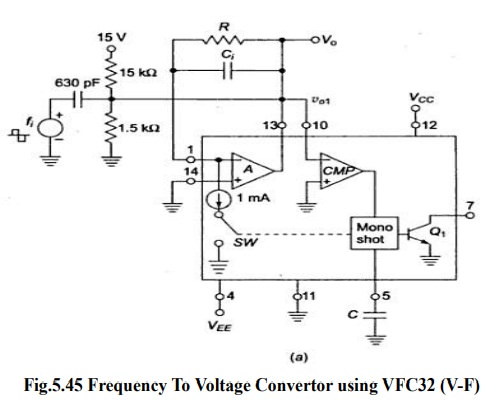Home | | Linear Integrated Circuits | Frequency to Voltage (F-V) and voltage to frequency convertors (V-F)

# Frequency to Voltage (F-V) and voltage to frequency convertors (V-F)

F-V convertors applications: Tachometer in motor speed control Rotational speed measurement.

Frequency to Voltage (F-V) and voltage to frequency convertors (V-F)

## Frequency to Voltage convertors (F-V)

┬Ę           F-V convertors applications: Tachometer in motor speed control Rotational speed measurement.

┬Ę           Two types of it: Pulse integrating Phase locked loop┬Ę           F-V convertor produces an output voltage whose amplitude is a function of input signal frequency.

┬Ę           V0=kf fi   kf is sensitivity of F-V convertor

┬Ę           It is basically a FM discriminator.Input frequency is applied to comparator A.

Resistor R acts as feedback element.

Capacitor Ci enables charge-balancing,

High pass network conditions input signal

For negative spike of V 01, comparator COMP triggers one shot multivibrator with threshold 7.5V The output of multivibrator closes the switch SW, for a time TH, this causes voltage Vo to build up and inject thru R and this continues until current out of summing input of opamp is equal to that injected by Vo through R continuously.

Vo=10-3 *TH *R*fi           as TH =7.5 C /1X10-3

Ripple Voltage,  Vr(max) =7.5 C /Ci

## Voltage to frequency convertor

Principle: Charge balancing technique-the process of charging and discharging results in frequency proportional to input signal F0= k Vi

Operation: Op-amp A converts input Vi to current Ii = Vi/R into summing junction.

When switch SW is open the current flows into capacitor Ci and charges it, and node voltage Vo1 produce ramp down.

When V01 =0 CMP triggers and sends a triggering signal to one shot multivibrator that closes the switch SW and turns transistor Q ON for time TH.

The threshold of mono shot = 7.5 V and TH= 7.5 C/10-3

During TH  , V01 ramps upward by amount ŌłåV01=(1mA-Ii) TH /Ci

Time duration TL for vo1 to return to 0 is TL = CŌłåV01/Ii

TL+TH = 1mA TH /Ii   = T

F0=Vi/7.5 RCTags : Ideal characteristics, Principle, Operation , Linear Integrated Circuits : Waveform Generators and Special Function ICs
Study Material, Lecturing Notes, Assignment, Reference, Wiki description explanation, brief detail
Linear Integrated Circuits : Waveform Generators and Special Function ICs : Frequency to Voltage (F-V) and voltage to frequency convertors (V-F) | Ideal characteristics, Principle, Operation